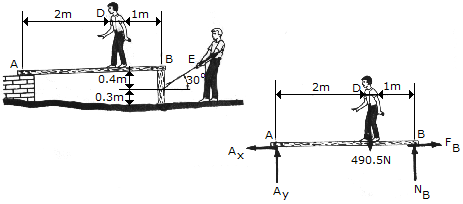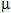# Engineering Mechanics - Friction - Discussion

### Discussion :: Friction - General Questions (Q.No.1)

1.The boy at D has a mass of 50 kg, a center of mass at G, and stands on a plank at the position shown. The plank is pin-supported at A and rests on a post at B. Neglecting the weight of the plank and post, determine the magnitude of force P his friend (?) at E must exert in order to pull out the post. TakeB = 0.3 andC = 0.8.

 [A]. P = 360 N [B]. P = 264 N [C]. P = 229 N [D]. P = 293 N

Explanation:

No answer description available for this question.

 Aswathvignesh said: (Jun 10, 2011) How to solve this problem?

 Mani said: (Jun 16, 2011) Ya how to solve the problem?

 Swapnil Dafal said: (Oct 16, 2011) Draw the free body digram (FBD). Then take sammetion of fx & sammetion of fy equal to zero and find moment with respect to point a. Also apply work energy principle for bettting third equation.

 Vignesh said: (Mar 28, 2012) Here should we take planck and post as two bodies and draw the free body diagrams for each of them? please explain the procedure!

 Rohan said: (Apr 9, 2012) Draw fbd of the diagram then calculate summation of x and why separately also take moment at point a.

 Nasma Noor N V said: (Mar 26, 2014) I am getting two different answers if we take first fbd of plank and then post. If we take moment about the base for post am getting 264N. If I take sum of forces in x and why direction am getting 284. 1.

 Alfredo said: (May 25, 2015) I need more explanation to your solution thank you and regard.

 Lira said: (Jun 1, 2015) I still don't understand how do you get into the answer?

 Hasan said: (Jun 4, 2015) I want the details of solution?

 Madara said: (Apr 12, 2016) Explain the solving method of this question.

 Sumit Manna said: (Jul 23, 2016) Force create at A=50g/3 and force in B = 100g/3 verticaly both Friction force is 0.3 * 100g/3 at B. Moment about C : 0.3 * 100g/3 * (0.3 + 0.4) = Fcos30 * 0.3. So F= 264N.

 James Oketch said: (Feb 13, 2017) Not able to reach the answer. Please explain me in detail.

 Debolina said: (Aug 26, 2017) Please solve it clearly.

 Shree said: (Oct 23, 2018) Please explain in detail @Sumit manna.

 Ajay Kumar said: (May 13, 2019) Please solve it.

 Chethan said: (Jul 4, 2020) Take vertical forces equal to zero, Ay + Ny = 490.5. Take moment at A, then Ny*3=mg*2, By solving we get N=327. Friction force = static friction coefficient * normal force. f = 0.3 * 327 = 98.1; Now take moment at c(post bottom),we get f(0.3+0.4) = Fcos30 * 0.3 By solving the above equation we get F = 264.115 Newtons.